# sfepy.discrete.fem.lcbc_operators module¶

Operators for enforcing linear combination boundary conditions in nodal FEM setting.

class sfepy.discrete.fem.lcbc_operators.EdgeDirectionOperator(name, regions, dof_names, dof_map_fun, filename, variables, ts=None, functions=None)[source]

Transformation matrix operator for edges direction LCBCs.

The substitution (in 3D) is: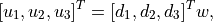whereis an edge direction vector averaged into a node. The new DOF is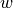.

get_vectors(nodes, region, field, filename=None)[source]
kind = 'edge_direction'
class sfepy.discrete.fem.lcbc_operators.IntegralMeanValueOperator(name, regions, dof_names, dof_map_fun, variables, ts=None, functions=None)[source]

Transformation matrix operator for integral mean value LCBCs. All DOFs in a region are summed to form a single new DOF.

kind = 'integral_mean_value'
class sfepy.discrete.fem.lcbc_operators.LCBCOperator(name, regions, dof_names, dof_map_fun, variables, functions=None)[source]

Base class for LCBC operators.

setup()[source]
class sfepy.discrete.fem.lcbc_operators.LCBCOperators(name, variables, functions=None)[source]

Container holding instances of LCBCOperator subclasses for a single variable.

Create a new LCBC operator described by bc, and add it to the container.

Parameters
bcLinearCombinationBC instance

The LCBC condition description.

tsTimeStepper instance

The time stepper.

append(op)[source]
finalize()[source]

Call this after all LCBCs of the variable have been added.

Initializes the global column indices and DOF counts.

Assemble all LCBC operators into a single matrix.

Parameters

The active DOF information.

new_onlybool

If True, the operator columns will contain only new DOFs.

Returns
mtx_lccsr_matrix

The global LCBC operator in the form of a CSR matrix.

rhs_lcarray

The right-hand side for non-homogeneous LCBCs.

lcdiDofInfo

The global active LCBC-constrained DOF information.

class sfepy.discrete.fem.lcbc_operators.MRLCBCOperator(name, regions, dof_names, dof_map_fun, variables, functions=None)[source]

Base class for model-reduction type LCBC operators.

These operators are applied to a single field, and replace its DOFs in a given region by new DOFs. In case some field DOFs are to be preserved, those have to be “copied” explicitly, by setting the corresponding row of the operator matrix to a single value one (see, for example, NoPenetrationOperator).

setup()[source]
treat_pbcs(dofs, master)[source]

Treat dofs with periodic BC.

class sfepy.discrete.fem.lcbc_operators.NoPenetrationOperator(name, regions, dof_names, dof_map_fun, filename, variables, ts=None, functions=None)[source]

Transformation matrix operator for no-penetration LCBCs.

kind = 'no_penetration'
class sfepy.discrete.fem.lcbc_operators.NodalLCOperator(name, regions, dof_names, dof_map_fun, constraints, variables, ts=None, functions=None)[source]

Transformation matrix operator for the general linear combination of DOFs in each node of a field in the given region.

The DOFs can be fully constrained - then the operator corresponds to enforcing Dirichlet boundary conditions.

The linear combination is given by:where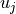,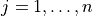are the DOFs in the node and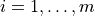,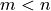, are the linear constraint indices.

SymPy is used to solve the constraint linear system in each node for the dependent DOF(s).

kind = 'nodal_combination'
class sfepy.discrete.fem.lcbc_operators.NormalDirectionOperator(name, regions, dof_names, dof_map_fun, filename, variables, ts=None, functions=None)[source]

Transformation matrix operator for normal direction LCBCs.

The substitution (in 3D) is: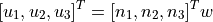The new DOF is.

get_vectors(nodes, region, field, filename=None)[source]
kind = 'normal_direction'
class sfepy.discrete.fem.lcbc_operators.RigidOperator(name, regions, dof_names, dof_map_fun, variables, ts=None, functions=None)[source]

Transformation matrix operator for rigid LCBCs.

kind = 'rigid'
class sfepy.discrete.fem.lcbc_operators.ShiftedPeriodicOperator(name, regions, dof_names, dof_map_fun, shift_fun, variables, ts, functions)[source]

Transformation matrix operator for shifted periodic boundary conditions.

This operator ties existing DOFs of two fields in two disjoint regions together. Unlike MRLCBCOperator subclasses, it does not create any new DOFs.

kind = 'shifted_periodic'